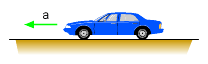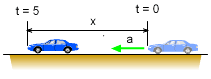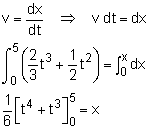Ch 1. Particle General Motion Multimedia Engineering Dynamics Position,Vel & Accel. Accel. varyw/ Time Accel. Constant Rect. Coordinates Norm/Tang. Coordinates Polar Coordinates RelativeMotion
 Chapter - Particle - 1. General Motion 2. Force & Accel. 3. Energy 4. Momentum - Rigid Body - 5. General Motion 6. Force & Accel. 7. Energy 8. Momentum 9. 3-D Motion 10. Vibrations Appendix Basic Math Units Basic Equations Sections Search eBooks Dynamics Fluids Math Mechanics Statics Thermodynamics Author(s): Kurt Gramoll ©Kurt GramollDYNAMICS - EXAMPLE ExampleCar Acceleration The acceleration of a car over time is know as a(t) = (2t2 + t) m/s2. Assuming the car starts from rest, how far does the car travel in 5 seconds? SolutionCar at t = 0 and t = 5 seconds. Since distance is needed, the acceleration equation must be integrated twice. First, find the velocity function,Notice, the integration was done with time, t, and not 5 seconds. Since the velocity at 5 seconds is not requested, only the general velocity function is determined. Next, find the distance traveled in 5 seconds by integrating the velocity function that was just determined,The final position after 5 seconds is x = (625 + 125) / 6 = 125 m

Practice Homework and Test problems now available in the 'Eng Dynamics' mobile app
Includes over 400 problems with complete detailed solutions.
Available now at the Google Play Store and Apple App Store.